• 用于单变量线性回归测试的一个数据集。单变量线性回归的代码在另一篇博文中可见。
• 单变量线性回归


TensorFlow笔记（4） 单变量线性回归

1. 单变量的线性方程
2. 准备数据
3. 构建模型
4. 训练模型
5. 模型预测

1. 单变量的线性方程
单变量的线性方程可以表示为  y = w ∗ x + b y = w * x +b 
展开全文深度学习
• 今天小编就为大家分享一篇python实现简单的单变量线性回归方法，具有很好的参考价值，希望对大家有所帮助。一起跟随小编过来看看吧
• 单变量线性回归可视化演示pathon版本，包含ex1data1数据文件完整调试版
• 线性回归分为单变量线性回归、多变量线性回归 区别 多变量比变量多个特征缩放 代码及注释 import numpy as np import matplotlib.pyplot as plt # 中文、负号 plt.rcParams['font.sans-serif'] = ['SimHei'] plt....
分析
线性回归分为单变量线性回归、多变量线性回归 区别 多变量比单变量多个特征缩放
代码及注释
import numpy as np
import matplotlib.pyplot as plt

# 中文、负号
plt.rcParams['font.sans-serif'] = ['SimHei']
plt.rcParams['axes.unicode_minus'] = False

# 读取数据

# 洗牌
np.random.seed(5)
np.random.permutation(data)

# 提取数据
x,y = data[:,:-1],data[:,-1]

# 数据预处理
def preProcess(x,y):
#特征缩放
x -= np.mean(x,0)
x /= np.std(x,0,ddof=1)

# 数据初始化
x = np.c_[np.ones(len(x)),x]
y = np.c_[y]
return x,y
x,y = preProcess(x,y)

# 切分
train_x,test_x = np.split(x,[int(0.7*len(x))])
train_y,test_y = np.split(y,[int(0.7*len(x))])

# 模型
def model(x,theta):
h = np.dot(x,theta)
return h

# 代价函数
def costFunc(h,y):
e = h - y
j = (1/(2*len(y)))*np.dot(e.T,e)
return j

# 梯度下降函数
m,n = x.shape

# 初始化
theta = np.zeros((n,1))
j_history = np.zeros(max_iter)

for i in range(max_iter):
h = model(x,theta)
j_history[i] = costFunc(h,y)

deltatheta = (1/m)*np.dot(x.T,h-y)
theta -= deltatheta*alpha
return j_history,theta
print(theta)

plt.title('代价函数图像')
plt.plot(j_history)
plt.show()

# 测试值
train_h = model(train_x,theta)
test_h = model(test_x,theta)

# 精度
def score(h,y):
u = np.sum(np.square(h-y))
v = np.sum(np.square(y-np.mean(y)))
return 1-u/v

print('训练集精度:',score(train_h,train_y))
print('测试集精度:',score(test_h,test_y))

plt.title('真实值与测试值对比图')
plt.scatter(train_y,train_y,label='真实值')
plt.scatter(train_y,train_h,label='测试值')
plt.legend()
plt.show()


效果展示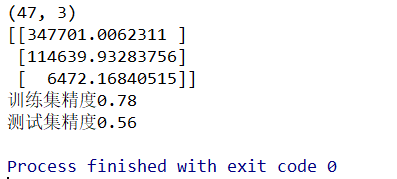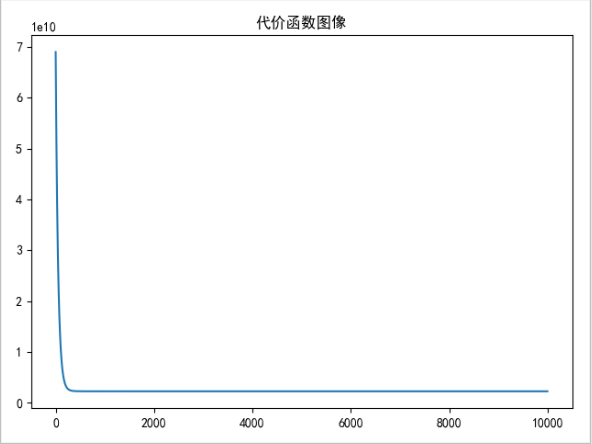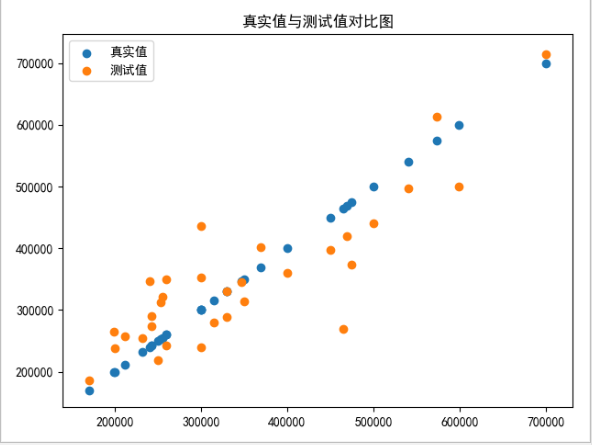展开全文机器学习 有监督学习
• 单变量线性回归梯度下降ipynb代码，根据Andrew课程的资料和数据码的算法代码
• 文章目录第2章：单变量线性回归2.1 模型描述 Model representation2.2 代价函数 Cost function2.3 梯度下降 Gradient descent2.4 线性回归的梯度下降 Gradient descent for linear regression 第2章：单变量线性回归...
• 单变量线性回归 import numpy as np import pandas as pd import matplotlib.pyplot as plt path = 'ex1data1.txt' data = pd.read_csv(path, header=None, names=['Population', 'Profit']) data.head()...
机器学习练习 1 - 线性回归
单变量线性回归
import numpy as np
import pandas as pd
import matplotlib.pyplot as plt

path =  'ex1data1.txt'


PopulationProfit06.110117.592015.52779.130228.518613.662037.003211.854045.85986.8233
data.describe()


PopulationProfitcount97.00000097.000000mean8.1598005.839135std3.8698845.510262min5.026900-2.68070025%5.7077001.98690050%6.5894004.56230075%8.5781007.046700max22.20300024.147000
看下数据长什么样子
data.plot(kind='scatter', x='Population', y='Profit', figsize=(12,8))
plt.show()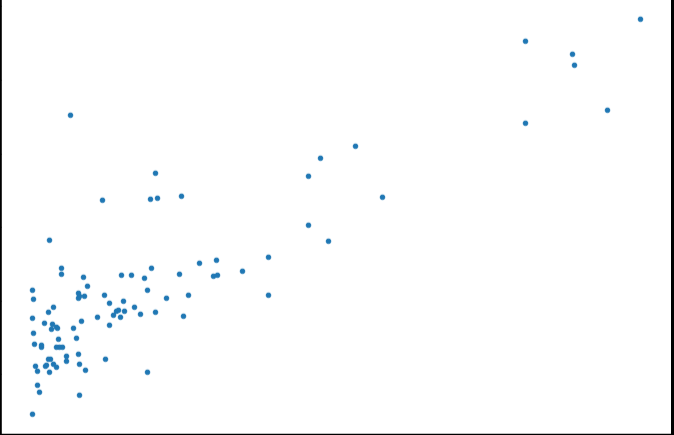现在让我们使用梯度下降来实现线性回归，以最小化成本函数。 以下代码示例中实现的方程在“练习”文件夹中的“ex1.pdf”中有详细说明。
首先，我们将创建一个以参数θ为特征函数的代价函数

J

(

θ

)

=

1

2

m

∑

i

=

1

m

(

h

θ

(

x

(

i

)

)

−

y

(

i

)

)

2

J\left( \theta \right)=\frac{1}{2m}\sum\limits_{i=1}^{m}{{{\left( {{h}_{\theta }}\left( {{x}^{(i)}} \right)-{{y}^{(i)}} \right)}^{2}}}

其中：${{h}{\theta }}\left( x \right)={{\theta }^{T}}X={{\theta }{0}}{{x}{0}}+{{\theta }{1}}{{x}{1}}+{{\theta }{2}}{{x}{2}}+…+{{\theta }{n}}{{x}_{n}}$
def computeCost(X, y, theta):
inner = np.power(((X * theta.T) - y), 2)
return np.sum(inner) / (2 * len(X))

让我们在训练集中添加一列，以便我们可以使用向量化的解决方案来计算代价和梯度。
data.insert(0, 'Ones', 1)

现在我们来做一些变量初始化。
# set X (training data) and y (target variable)
cols = data.shape
X = data.iloc[:,0:cols-1]#X是所有行，去掉最后一列
y = data.iloc[:,cols-1:cols]#X是所有行，最后一列

观察下 X (训练集) and y (目标变量)是否正确.
X.head()#head()是观察前5行


OnesPopulation016.1101115.5277218.5186317.0032415.8598
y.head()


Profit017.592019.1302213.6620311.854046.8233
代价函数是应该是numpy矩阵，所以我们需要转换X和Y，然后才能使用它们。 我们还需要初始化theta。
X = np.matrix(X.values)
y = np.matrix(y.values)
theta = np.matrix(np.array([0,0]))

theta 是一个(1,2)矩阵
theta

matrix([[0, 0]])

看下维度
X.shape, theta.shape, y.shape

((97, 2), (1, 2), (97, 1))

计算代价函数 (theta初始值为0).
computeCost(X, y, theta)

32.072733877455676


θ

j

:

=

θ

j

−

α

∂

∂

θ

j

J

(

θ

)

{{\theta }_{j}}:={{\theta }_{j}}-\alpha \frac{\partial }{\partial {{\theta }_{j}}}J\left( \theta \right)

def gradientDescent(X, y, theta, alpha, iters):
temp = np.matrix(np.zeros(theta.shape))
parameters = int(theta.ravel().shape)
cost = np.zeros(iters)

for i in range(iters):
error = (X * theta.T) - y

for j in range(parameters):
term = np.multiply(error, X[:,j])
temp[0,j] = theta[0,j] - ((alpha / len(X)) * np.sum(term))

theta = temp
cost[i] = computeCost(X, y, theta)

return theta, cost

初始化一些附加变量 - 学习速率α和要执行的迭代次数。
alpha = 0.01
iters = 1000

现在让我们运行梯度下降算法来将我们的参数θ适合于训练集。
g, cost = gradientDescent(X, y, theta, alpha, iters)
g

matrix([[-3.24140214,  1.1272942 ]])

最后，我们可以使用我们拟合的参数计算训练模型的代价函数（误差）。
computeCost(X, y, g)

4.5159555030789118

现在我们来绘制线性模型以及数据，直观地看出它的拟合。
x = np.linspace(data.Population.min(), data.Population.max(), 100)
f = g[0, 0] + (g[0, 1] * x)

fig, ax = plt.subplots(figsize=(12,8))
ax.plot(x, f, 'r', label='Prediction')
ax.scatter(data.Population, data.Profit, label='Traning Data')
ax.legend(loc=2)
ax.set_xlabel('Population')
ax.set_ylabel('Profit')
ax.set_title('Predicted Profit vs. Population Size')
plt.show()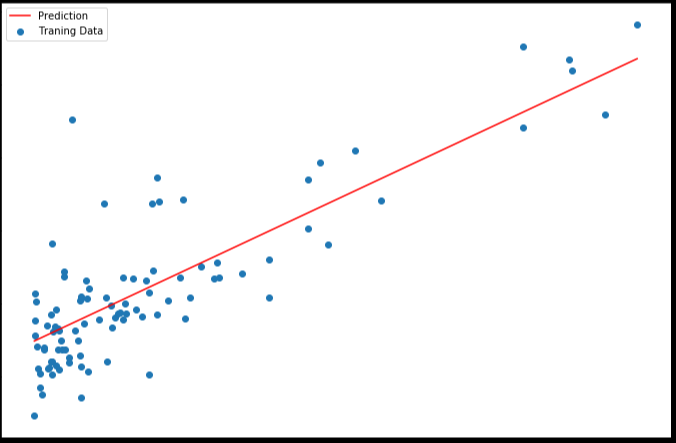由于梯度方程式函数也在每个训练迭代中输出一个代价的向量，所以我们也可以绘制。 请注意，代价总是降低 - 这是凸优化问题的一个例子。
fig, ax = plt.subplots(figsize=(12,8))
ax.plot(np.arange(iters), cost, 'r')
ax.set_xlabel('Iterations')
ax.set_ylabel('Cost')
ax.set_title('Error vs. Training Epoch')
plt.show()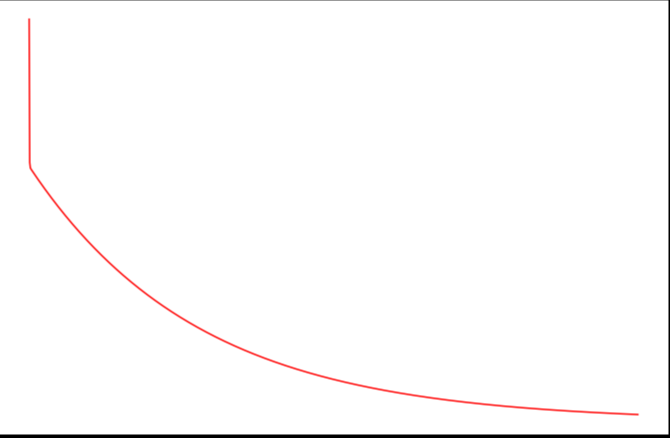多变量线性回归
练习1还包括一个房屋价格数据集，其中有2个变量（房子的大小，卧室的数量）和目标（房子的价格）。 我们使用我们已经应用的技术来分析数据集。
path =  'ex1data2.txt'


SizeBedroomsPrice021043399900116003329900224003369000314162232000430004539900
对于此任务，我们添加了另一个预处理步骤 - 特征归一化。 这个对于pandas来说很简单
data2 = (data2 - data2.mean()) / data2.std()


SizeBedroomsPrice00.130010-0.2236750.4757471-0.504190-0.223675-0.08407420.502476-0.2236750.2286263-0.735723-1.537767-0.86702541.2574761.0904171.595389
现在我们重复第1部分的预处理步骤，并对新数据集运行线性回归程序。
# add ones column
data2.insert(0, 'Ones', 1)

# set X (training data) and y (target variable)
cols = data2.shape
X2 = data2.iloc[:,0:cols-1]
y2 = data2.iloc[:,cols-1:cols]

# convert to matrices and initialize theta
X2 = np.matrix(X2.values)
y2 = np.matrix(y2.values)
theta2 = np.matrix(np.array([0,0,0]))

# perform linear regression on the data set
g2, cost2 = gradientDescent(X2, y2, theta2, alpha, iters)

# get the cost (error) of the model
computeCost(X2, y2, g2)

0.13070336960771892

我们也可以快速查看这一个的训练进程。
fig, ax = plt.subplots(figsize=(12,8))
ax.plot(np.arange(iters), cost2, 'r')
ax.set_xlabel('Iterations')
ax.set_ylabel('Cost')
ax.set_title('Error vs. Training Epoch')
plt.show()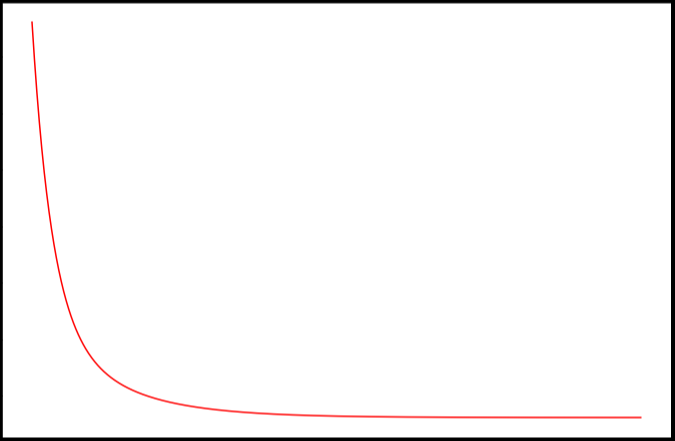我们也可以使用scikit-learn的线性回归函数，而不是从头开始实现这些算法。 我们将scikit-learn的线性回归算法应用于第1部分的数据，并看看它的表现。
from sklearn import linear_model
model = linear_model.LinearRegression()
model.fit(X, y)

LinearRegression(copy_X=True, fit_intercept=True, n_jobs=1, normalize=False)

scikit-learn model的预测表现
x = np.array(X[:, 1].A1)
f = model.predict(X).flatten()

fig, ax = plt.subplots(figsize=(12,8))
ax.plot(x, f, 'r', label='Prediction')
ax.scatter(data.Population, data.Profit, label='Traning Data')
ax.legend(loc=2)
ax.set_xlabel('Population')
ax.set_ylabel('Profit')
ax.set_title('Predicted Profit vs. Population Size')
plt.show()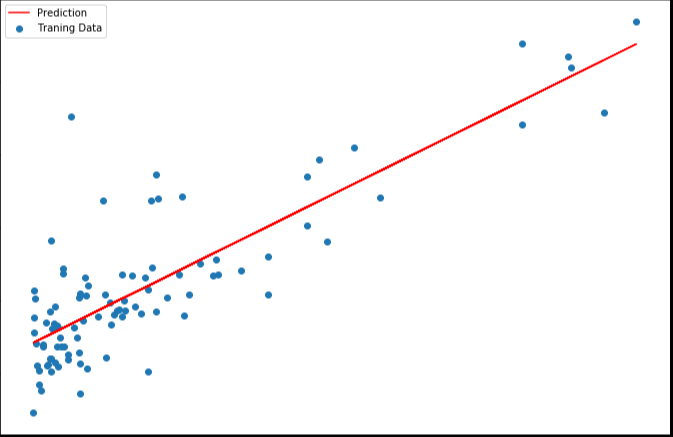4. normal equation（正规方程）
正规方程是通过求解下面的方程来找出使得代价函数最小的参数的：

∂

∂

θ

j

J

(

θ

j

)

=

0

\frac{\partial }{\partial {{\theta }_{j}}}J\left( {{\theta }_{j}} \right)=0

。 假设我们的训练集特征矩阵为 X（包含了

x

0

=

1

{{x}_{0}}=1

）并且我们的训练集结果为向量 y，则利用正规方程解出向量

θ

=

(

X

T

X

)

−

1

X

T

y

\theta ={{\left( {{X}^{T}}X \right)}^{-1}}{{X}^{T}}y

。 上标T代表矩阵转置，上标-1 代表矩阵的逆。设矩阵

A

=

X

T

X

A={{X}^{T}}X

，则：

(

X

T

X

)

−

1

=

A

−

1

{{\left( {{X}^{T}}X \right)}^{-1}}={{A}^{-1}}

梯度下降与正规方程的比较：
梯度下降：需要选择学习率α，需要多次迭代，当特征数量n大时也能较好适用，适用于各种类型的模型
正规方程：不需要选择学习率α，一次计算得出，需要计算

(

X

T

X

)

−

1

{{\left( {{X}^{T}}X \right)}^{-1}}

，如果特征数量n较大则运算代价大，因为矩阵逆的计算时间复杂度为

O

(

n

3

)

O(n3)

，通常来说当

n

n

小于10000 时还是可以接受的，只适用于线性模型，不适合逻辑回归模型等其他模型
# 正规方程
def normalEqn(X, y):
theta = np.linalg.inv(X.T@X)@X.T@y#X.T@X等价于X.T.dot(X)
return theta

final_theta2=normalEqn(X, y)#感觉和批量梯度下降的theta的值有点差距
final_theta2

matrix([[-3.89578088],
[ 1.19303364]])

#梯度下降得到的结果是matrix([[-3.24140214,  1.1272942 ]])

在练习2中，我们将看看分类问题的逻辑回归。


展开全文• python3.5纯生代码实现单变量线性回归预测直线y=mx+b，不调用机器学习第三方库，仅调用numpy实现矩阵向量运算以及matplotlib实现画图。参数更新方法为梯度下降法。
• Tensorflow实现梯度下降的单变量线性回归模型，使用到的库：TensorFlow、Numpy、Matplotlib
• matlab开发-单变量线性回归梯度描述算法。一种实用的机器学习算法
• tensorflow单变量线性回归（一次函数拟合）的jupyter笔记
• ## 单变量线性回归

千次阅读 2016-04-08 12:40:51
单变量线性回归就是从一个输入值预测一个输出值。输入/输出关系是一个线性函数。 模型表示： 我们用一个根据房屋尺寸预测房屋售价的例子开始。 假设有一个数据集，我们称作训练集，如下表所示 我们用来描述回归...
单变量线性回归就是从一个输入值预测一个输出值。输入/输出关系是一个线性函数。
模型表示：

我们用一个根据房屋尺寸预测房屋售价的例子开始。

假设有一个数据集，我们称作训练集，如下表所示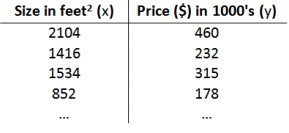我们用来描述回归问题的标记如下：

m 代表训练集中实例的数量

x 代表特征/输入变量

y 代表目标变量/输出变量

(x,y) 代表训练集中的实例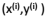代表第i个观测实例

h 代表学习算法的函数也称为假设（hypothesis）

然后我们看一下监督学习的工作方式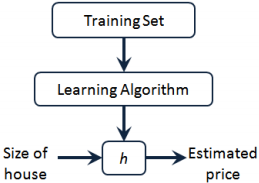我们把训练集（房屋尺寸）作为输入喂给学习算法，学习算法训练后输出一个函数
（h），通过这个函数我们可以预测房屋售价。

假设房屋尺寸与房屋售价是线性关系，我们假设函数（hypothesis function）为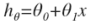，因为只有一个特征/输入变量，所以这样的问题叫做单变量线性回归问题。

代价函数（cost function）：

现在我们有了假设函数，接下来要做的就是选择合适的参数（parameter）
Θ。

我们的目标是用假设函数进行预测，因此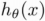要最接近
y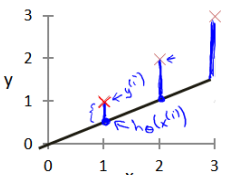因此我们引出代价函数（cost function）也叫平方误差代价函数：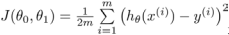，其中½是为了接下来的计算方便。

我们的目标就是找出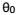和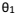使得代价函数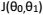最小。

我们绘制一个等高线图，三个坐标分别为、和：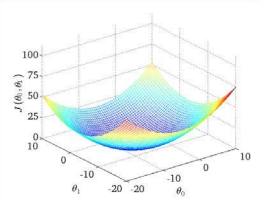我们可以看到存在一个点使得最小。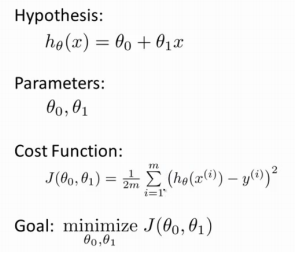现在我们看看代价函数是干什么的。

当=0时，与随着变化如下：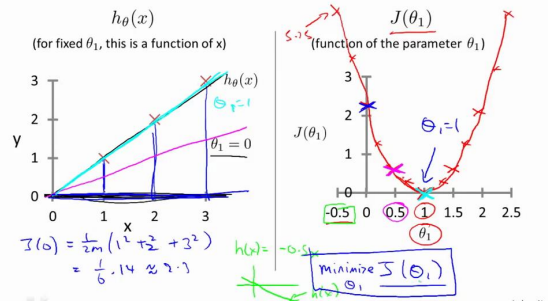在=1时，取得最小值，与y最接近。

正如我们上面提到的，当与均不为0时，存在一个点使得最小，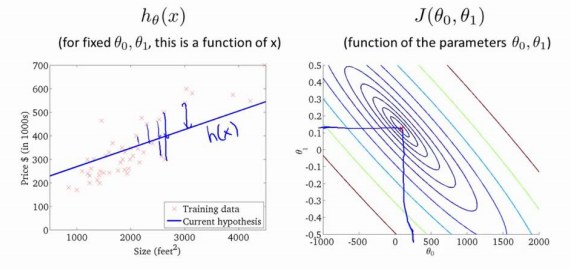现在我们知道了代价函数，那么我们接下来就要一个有效的算法，自动地找出使代价函数最小的参数和。

梯度下降是一个用来计算函数最小值的算法，所以我们要使用梯度下降算法来求出代价函数的最小值。

梯度下降的基本思想是：开始随机定义一个参数组合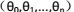，然后不断地改变参数的值，使得每次代价函数J下降最多，直到找到一个局部最小值。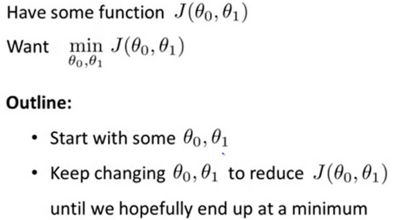想象一下，你现在站在山上一个点，然后运用梯度下降观察周围，找到下降最快的方向，并朝着这个方向下山。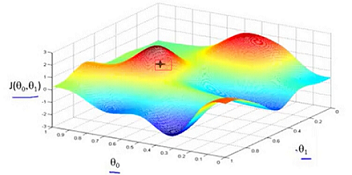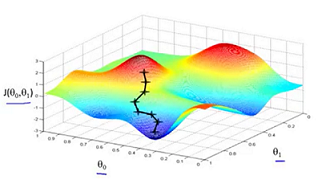当然，如果你的起始点不同，你所走的路径和找到的最低点也可能不同。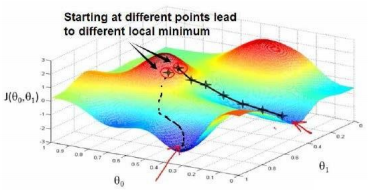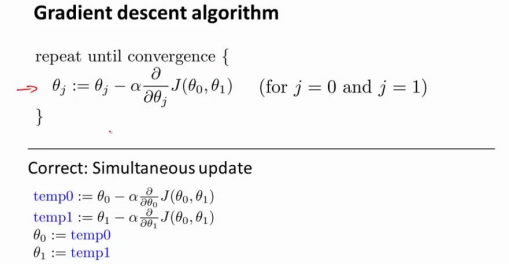其中，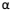是学习率（learning rate），就是梯度下降每次下降的步长（学习速率），需要注意的是，我们要同时更新和。

下面是梯度下降的直观理解：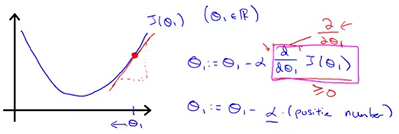减去红线斜率（正数），所以减小，直到红线斜率等于0，不变，J最小；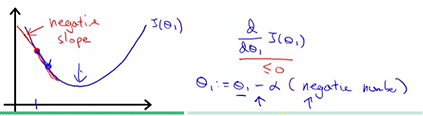另一方向同理，减去红线斜率（负数），增大，直到斜率为0，不变，J最小。

如果学习率太小，那么下降速度比较慢，而如果学习率太大，梯度下降可能无法收敛。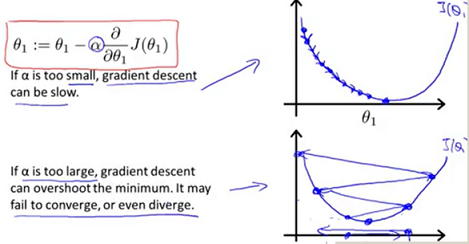如果一开始参数选择在局部最优点，那么这个点的导数为0，参数
Θ不会更新。

在梯度下降的过程中，导数的值会不断变小，下降幅度也会减小，因此在下降过程中没有必要对学习率进行调整。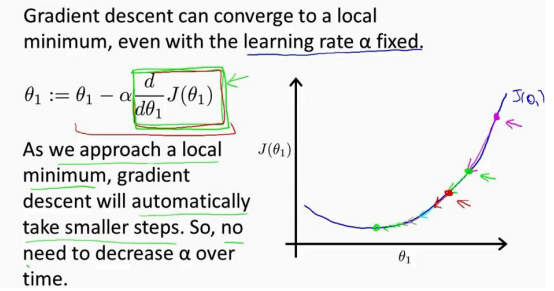梯度下降的线性回归：

现在我们将梯度下降和代价函数结合。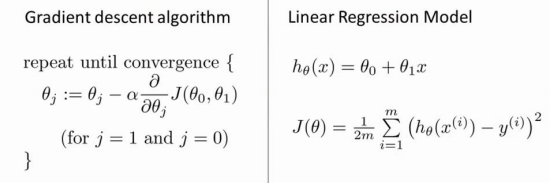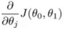表示代价函数对和分别求导：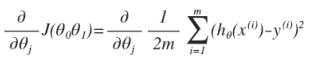j=0时：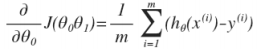j=1时：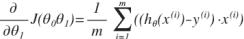所以算法改写成：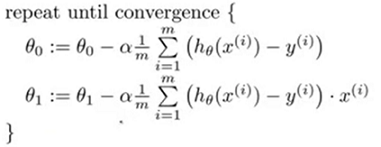这就是线性代数的梯度下降法。

下面是线性分类与线性回归比较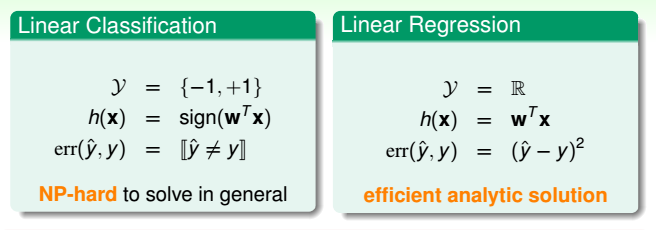参考资料：Machine Learning——Andrew Ng (Coursera)

机器学习基石——林轩田 (Coursera)

展开全文数据挖掘 机器学习
• tensorflow单变量线性回归（一次函数拟合） 本文章为本人自己写的jupyter笔记，图片显示有一点问题（下面的每个图片都变成同一张图片），所以仅供预览。 需要下载ipynb文件的朋友可以关注我并找到我上传的资源。 ....tensorflow
• 摘要： 给出一个房价预测的例子，x轴是房子的大小，y轴是房子的价格，图中标注了一些房子作为数据集，而这些点被称为标注数据(labeled data)，利用这样的...Linear Regression with one Variable(单变量线性
• 线性回归 线性回归（Linear Regression)作为Machine Learning 整个课程的切入例子确实有独到的...线性回归可以分为单变量线性回归(Linear Regression with One Variable)以及多变量线性回归(Linear Regression wi
• 1.0.单变量线性回归 1）题目： 在本部分的练习中，您将使用一个变量实现线性回归，以预测食品卡车的利润。假设你是一家餐馆的首席执行官，正在考虑不同的城市开设一个新的分店。该连锁店已经在各个城市拥有卡车，...机器学习 吴恩达
• 本人通过观看吴恩达机器学习视频所记录的笔记，比较潦草，有兴趣的可以看一看。 知识点概要： 1、假设函数 2、代价函数 3、梯度下降法 4、如何选择学习率 5、关于线性回归的梯度下降
• 【Linear Regression with One Variable】1. 线性回归模型单变量线性回归是通过给出的样本变量与输出，预测一个变量的对应输出，属于监督学习。
• 线性回归是机器学习中的基础算法之一，属于监督学习中的回归问题，...这里主要使用python的sklearn实现一个简单的单变量线性回归。 sklearn对机器学习方法封装的十分好，基本使用fit,predict,score，来训练，预测，评价python 机器学习
• Stanford机器学习课程笔记——单变量线性回归和梯度下降法   1. 问题引入  单变量线性回归就是我们通常说的线性模型，而且其中只有一个自变量x，一个因变量y的那种最简单直接的模型。模型的数学表达式为y=...梯度下降法 代价函数
• 目录 0. 前言 1. 假设函数（Hypothesis） 2. 代价函数（Cost Function） 3. 梯度下降（Gradient Descent） ...学习完吴恩达老师机器学习课程的单变量线性回归，简单的做个笔记...单变量线性回归（Linear Regr...机器学习 假设函数 代价函数 梯度下降
• Andrew线性回归算法的原始数据集 ，有相应的ipynb训练代码
• 其中Y为输出变量、响应变量、因变量、被解释变量；m为均值；e为不可控因子，可以理解为噪声。故模型等式右边是用X组成的函数去描述Y的均值，即模型是在平均的意义下去描述自变量与因变量间的关系...模型诊断 正态分布
• Coursera公开课笔记: 斯坦福大学机器学习第二课“单变量线性回归(Linear regression with one variable)” 发表于 2012年05月6号 由 52nlp 斯坦福大学机器学习第二课”单变量线性回归“学习笔记，本次课程...机器学习 笔记 coursera...